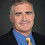## Wednesday, February 13, 2008

### Average Case versus Worst Case

A young theorist said something like this in a recent talk.
Complexity theorists studied worst-case complexity until the mid-80's and then have focused on average-case complexity since.
I asked him why he though that and he said that he had given an earlier talk and said complexity theorists focused only on worst-case complexity and was told this wasn't true since the mid-80's.

What did happen in the mid-80's? Levin came up with his definition of average-case complexity classes. Modern cryptography started to really grow around then with their emphasis on hard on average assumptions. Results like Nisan-Wigderson connected average-case hardness with derandomization.

But most of the great work in complexity continued to focus on worst-case complexity: Circuit lower bounds, nondeterministic space closed under complement, Toda's theorem, interactive proofs, PCPs, hardness of approximation results, Primes in P, SL=L and much more. Even derandomization results are now based on worst-case hardness.

Why the focus on worst instead of average case? We can't know the underlying distributions for sure and worst case complexity handles any distribution.

In crypto they know the underlying distribution since their protocols create it. But now even in that community you now see a slight move to base protocols on worst-case assumptions, using average-to-worst case reductions for lattice-based problems. I worry though that these average-to-worst case reductions imply less that the average case complexity of these problems are harder than we expected and more that the worst case is easier than we thought.

1.I think worse-case analysis helps to understand the problem, while average-case analysis helps to solve the problem.

2.Is "worst case complexity" supposed to appear twice in your quote? (If so, I don't understand how studying the same thing before and after the 80s made you think that something happened in the 80s.)

3.Whoops. Fixed.

4.why does worst case complexity cover any distribution? Can't the bad case occur with 0 measure?

5.From a non-theorist point of view, complexity results seem mainly useful as tools for suggesting whether a particular pproach to solving the problem is feasible. For example, it is quite valuable to know when solving an open problem at least whether it is NP-hard, NEXPTIME-hard, non-elementary, or undecidable.

My view of average case complexity was fundamentally shaken after learning that even undecidable problems like the group word problem can have low average case complexity.

6.I worry though that these average-to-worst case reductions imply less that the average case complexity of these problems are harder than we expected and more that the worst case is easier than we thought.

In a sense, this is exactly right, and even partially understood. For example, the shortest vector problem (SVP) is NP-hard (even to approximate to within any constant). But lattice-based crypto is based on the hardness of approximating SVP to within a factor of, say, n (the dimension of the lattice). This problem is known to be in coNP, so it's "easier" than exact-SVP. Is it still hard for poly-time algorithms? One assumes.....

7.8.kids say the darndest things

9.What examples do we have of problems that are easy from the average-case point of view but hard (provably or otherwise) from the smoothed-analysis point of view ?

10.What examples do we have of problems that are easy from the average-case point of view but hard (provably or otherwise) from the smoothed-analysis point of view

Despite appearing natural, this is not the right question. In both cases one needs a language or optimization problem and a distribution (in the average case on inputs, in the smoothed case on the perturbation). One can easily pick distributions that "separate" the two - for example, if both distributions are supported on single points the former refers to one input for each input size but the latter can become worst-case complexity.

11.I have always felt that a good distribution with respect to which one should measure average-case complexity, should come from an application. For example, a good input distribution for various congestion-minimizing routing algorithms
could be random preferential attachment graphs.

12.What examples do we have of problems that are easy from the average-case point of view but hard (provably or otherwise) from the smoothed-analysis point of view ?

Barany, Vempala, and Vetta proved that the average case complexity of two-player Nash equilibrium is polynomial. In contrast, Chen, Deng, Teng proved that if the smoothed complexity of two-player Nash Equilibrium is polynomial, then every PPAD problem can be solved in randomized polynomial-time.

13.A bottleneck in average-case analysis is that it is not sufficient to know the input distribution. Distributions need to be tracked throughout the computation. When composing two algorithms P1;P2 sequentially, for inputs from a collection I, the average time of the the composition is the sum of the average times of the programs, T(P1)(I) + TP2(P1(I)) where P1(I) is the output multi-set
produced by P1 on I. Even when the distribution of I is known, the distribution of P1(I) generally is not.

This made the exact average-case analysis of a number of algorithms, such as Heapsort, unknown or hard. A language in which operations support the tracking of data distributions (represented as random bags) in a modular
way, is discussed in
http://www.springer.com/computer/theoretical+computer+science/book/978-0-387-73383-8.

The approach led to new algorithms, such as Percolating Heapsort, addressing the open problem of the exact average time of Heapsort
variants. The language MOQA (MOdular Quantitative Analysis) and the
timing tool Distri-Track support static average-case analysis by tracking distributions and summing up of average-times of program components. Both worst-case and average-case can be derived for MOQA programs, including standard deviation and higher moments. Research is ongoing into linking smoothed analysis to this approach. www.ceol.ucc.ie